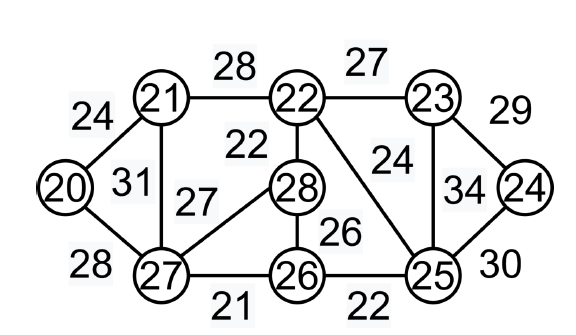# Kruskal's Minimum Spanning Tree Algorithm-Greedy algorithm in C++

A spanning tree is a linked and undirected graph subgraph that connects all vertices. Many spanning trees can exist in a graph. The minimum spanning tree (MST) on each graph is the same weight or less than all other spanning trees. Weights are assigned to edges of spanning trees and the sum is the weight assigned to each edge. As V is the number of vertices in the graph, the minimum spanning tree has edges of (V - 1), where V is the number of edges.

## Finding minimum spanning tree using Kruskal’s algorithm

• All of the edges should be arranged in a non-descending sequence of weight.

• Choose the smallest edge. This edge is included if the cycle is not formed.

• Step 2 should be performed until the spanning tree has (V-1) edges.

In this scenario, we are told to use a greedy method. The greedy option is to select the edge with the least amount of weight. As an illustration: The minimum spanning tree for this graph is (9-1)= 8 edges.After sorting:

Weight  Src    Dest
21       27    26
22       28    22
22       26    25
24       20    21
24       22    25
26       28    26
27       22    23
27       27    28
28       20    27
28       21    22
29       23    24
30       25    24
31       21    27
34       23    25

Now we need to pick all the edges according to the sort.

Edge 26-27-> included because no cycle is formed

Edge 28-22-> included because no cycle is formed

Edge 26-25-> included because no cycle is formed.

Edge 20-21-> included because no cycle is formed

Edge 22-25-> included because no cycle is formed.

Edge 28-26-> discarded as cycle is formed

Edge 22-23-> included because no cycle is formed

Edge 27-28-> discarded as cycle is formed

Edge 20-27-> included because no cycle is formed

Edge 21-22-> discarded as cycle is formed

Edge 23-24-> included because no cycle is formed

As the number of edges is (V-1), so the algorithm ends here.

## Example

#include <stdio.h>
#include <stdlib.h>
#include <string.h>
struct Edge {
int src, dest, weight;
};
struct Graph {
int V, E;
struct Edge* edge;
};
struct Graph* createGraph(int V, int E){
struct Graph* graph = (struct Graph*)(malloc(sizeof(struct Graph)));
graph->V = V;
graph->E = E;
graph->edge = (struct Edge*)malloc(sizeof( struct Edge)*E);
return graph;
}
struct subset {
int parent;
int rank;
};
int find(struct subset subsets[], int i){
if (subsets[i].parent != i)
subsets[i].parent
= find(subsets, subsets[i].parent);
return subsets[i].parent;
}
void Union(struct subset subsets[], int x, int y){
int xroot = find(subsets, x);
int yroot = find(subsets, y);
if (subsets[xroot].rank < subsets[yroot].rank)
subsets[xroot].parent = yroot;
else if (subsets[xroot].rank > subsets[yroot].rank)
subsets[yroot].parent = xroot;
else{
subsets[yroot].parent = xroot;
subsets[xroot].rank++;
}
}
int myComp(const void* a, const void* b){
struct Edge* a1 = (struct Edge*)a;
struct Edge* b1 = (struct Edge*)b;
return a1->weight > b1->weight;
}
void KruskalMST(struct Graph* graph){
int V = graph->V;
struct Edge
result[V];
int e = 0;
int i = 0;
qsort(graph->edge, graph->E, sizeof(graph->edge), myComp);
struct subset* subsets
= (struct subset*)malloc(V * sizeof(struct subset));
for (int v = 0; v < V; ++v) {
subsets[v].parent = v;
subsets[v].rank = 0;
}
while (e < V - 1 && i < graph->E) {
struct Edge next_edge = graph->edge[i++];
int x = find(subsets, next_edge.src);
int y = find(subsets, next_edge.dest);
if (x != y) {
result[e++] = next_edge;
Union(subsets, x, y);
}
}
printf("Following are the edges in the constructed MST\n");
int minimumCost = 0;
for (i = 0; i < e; ++i){
printf("%d -- %d == %d\n", result[i].src,
result[i].dest, result[i].weight);
minimumCost += result[i].weight;
}
printf("Minimum Cost Spanning tree : %d",minimumCost);
return;
}
int main(){
/* Let us create the following weighted graph
30
0--------1
| \       |
26| 25\ |15
| \ |
22--------23
24 */
int V = 24;
int E = 25;
struct Graph* graph = createGraph(V, E);
graph->edge.src = 20;
graph->edge.dest = 21;
graph->edge.weight = 30;
graph->edge.src = 20;
graph->edge.dest = 22;
graph->edge.weight = 26;
graph->edge.src = 20;
graph->edge.dest = 23;
graph->edge.weight = 25;
graph->edge.src = 21;
graph->edge.dest = 23;
graph->edge.weight = 35;
graph->edge.src = 22;
graph->edge.dest = 23;
graph->edge.weight = 24;
KruskalMST(graph);
return 0;
}

## Output

Following are the edges in the constructed MST
22 -- 23 == 24
20 -- 23 == 25
20 -- 21 == 30
Minimum Cost Spanning tree : 79

## Conclusion

This tutorial demonstrated how to use Kruskal's Minimum Spanning Tree Algorithm-Greedy method and C++ code to solve this problem. We can also write this code in java, python, and other languages. It was modelled by Kruskal's concept. This program finds the shortest spanning tree in a given graph. We hope you find this tutorial helpful.